# Monoidal transformation

(diff) ← Older revision | Latest revision (diff) | Newer revision → (diff)

blowing up,-process

A special kind of birational morphism of an algebraic variety or bimeromorphic morphism of an analytic space. For example, letbe an algebraic variety (or an arbitrary scheme), and let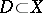be a closed subvariety given by a sheaf of ideals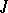. The monoidal transformation ofwith centreis the-scheme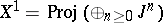— the projective spectrum of the graded sheaf of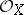-algebras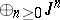. If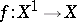is the structure morphism of the-scheme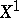, then the sheaf of ideals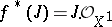on(defining the exceptional subscheme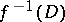on) is invertible. This means thatis a divisor on; in addition,induces an isomorphism betweenand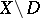. A monoidal transformationof a schemewith centreis characterized by the following universal property : The sheaf of ideals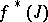is invertible and for any morphism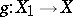for which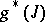is invertible there is a unique morphismsuch that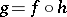.

A monoidal transformation of an algebraic or analytic spacewith as centre a closed subspacecan be defined and characterized in the same way.

An important class of monoidal transformations are the admissible monoidal transformations, which are distinguished by the condition thatis non-singular andis a normally flat scheme along. The latter means that all sheavesare flat-modules. The importance of admissible monoidal transformations is explained by the fact that they do not worsen the singularities of the variety. In addition, it has been proved (see ) that a suitable sequence of admissible monoidal transformations improves singularities, which allows one to prove the theorem on the resolution of singularities for an algebraic variety over a field of characteristic zero.

Admissible monoidal transformations of non-singular varieties are particularly simple to construct. If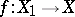is a monoidal transformation with a non-singular centre, then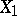is again non-singular and the exceptional subspaceis canonically isomorphic to the projectivization of the conormal sheaf toin. In the special case whenconsists of one point, the monoidal transformation consists of blowing up this point into the whole projective space of tangent directions. For the behaviour of various invariants of non-singular varieties (such as Chow rings, cohomology spaces, the-functor, and Chern classes) under admissible monoidal transformations see .

How to Cite This Entry:
Monoidal transformation. Encyclopedia of Mathematics. URL: http://encyclopediaofmath.org/index.php?title=Monoidal_transformation&oldid=13914
This article was adapted from an original article by V.I. Danilov (originator), which appeared in Encyclopedia of Mathematics - ISBN 1402006098. See original article